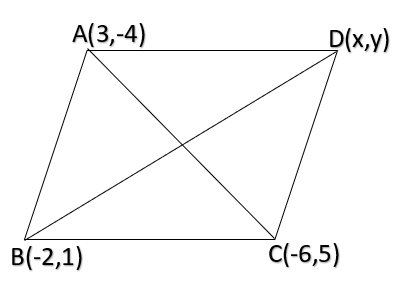QUESTION

# If (3, -4) and (-6,5) are the extremities of the diagonal of a parallelogram and (-2,1) is its third vertex then its fourth vertex isA. (-1,0)B. (0, -1)C. (-1,1)D. None of these

Hint: In this question, coordinates of three vertices of parallelogram are given, let the fourth coordinate be $(x,y)$. Calculate the coordinate for midpoint of diagonals from the known coordinate and equate it with unknown variables.Let A= (3, -4); C= (-6,5) as coordinate of diagonal AC
Let coordinate of D be $(x,y)$
Since diagonals of parallelogram bisect each other
Also, midpoint of $({x_1},{y_1})({x_2},{y_2}) = \left( {\dfrac{{{x_1} + {x_2}}}{2},\dfrac{{{y_1} + {y_2}}}{2}} \right) \\ \\$
Now, midpoint of AC = midpoint of BD
$\left( {\dfrac{{3 - 6}}{2},\dfrac{{ - 4 + 5}}{2}} \right) = \left( {\dfrac{{ - 2 + x}}{2},\dfrac{{1 + y}}{2}} \right) \\ \left( {\dfrac{{ - 3}}{2},\dfrac{1}{2}} \right) = \left( {\dfrac{{ - 2 + x}}{2},\dfrac{{1 + y}}{2}} \right) \\ {\text{Equating both sides}} \\$
$- 2 + x = - 3{\text{ and }}1 + y = 1 \\ x = - 1{\text{ and }}y = 0 \\$
Coordinates of fourth vertex is (-1,0)

Hence the correct answer is option A.

Note: For these types of questions, where we need to find the coordinates of any point on a parallelogram where 3 coordinates are already known, the best option is always to go through the midpoint approach, that is why it is always suggested to have a good knowledge of parallelograms and its diagonals. Also we can cross check this answer by comparing the slopes of the opposite lines, that is they should be the same.